Smartick is a fun way to learn math!Mar16

# Equivalent Fractions on a Number Line

Today we are going to learn how to solve activities related to the representation of fractions on a number line and the amplification and simplification of fractions. To do this we need to use equivalent fractions.

It is easy to represent a fraction on a number line when it is divided into the same number of units as the denominator.The line consists of one unit with 3 divisions. And since the denominator of the fraction is also 3, they match. In this case, it is easy to know that the marker must be placed in the second position on the number line in order to represent the fraction 2/3.

But it is not so simple when the unit on the number line is not divided into the same number as the denominator. One strategy is to match the two numbers. To do this, we must find equivalent fractions in which the denominator has the same number of divisions as the unit. We can do this by amplifying or simplifying a fraction.

#### Amplified Fractions on a Number Line

First, let’s learn how to represent a fraction on a number line when its unit has more divisions than the denominator of the fraction. In this case, the representation is not as obvious.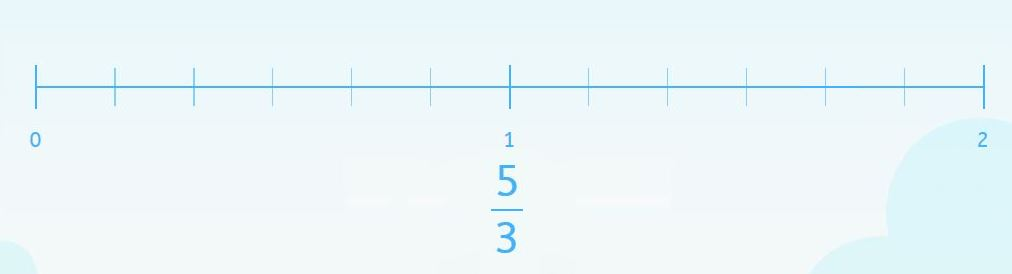On one hand, the number line has two units and each unit is divided into 6.

And on the other, the denominator of the fraction is 3.

They do not match and 6 is larger than 3.

We can look for an equivalent fraction. By amplifying the one we want to represent we can get a fraction with a denominator equal to the number of divisions in each unit on the number line. In this case, six.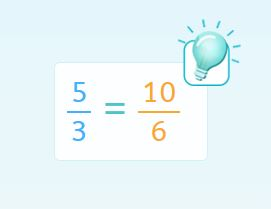Now we know that the marker should be in the tenth position on the number line.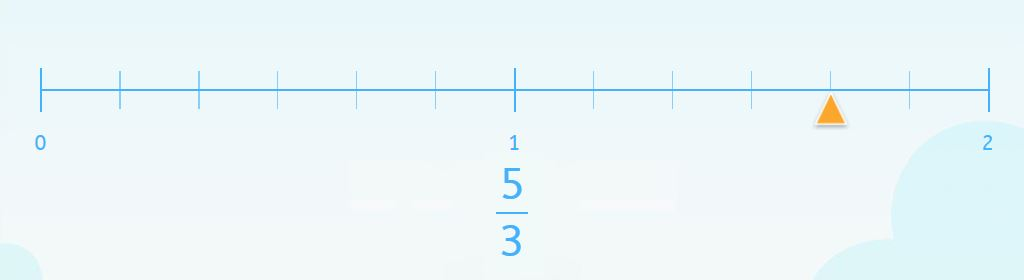#### Simplified Fractions on a Number Line

When the unit on the number line has fewer divisions than the denominator, we can use another similar strategy to represent it.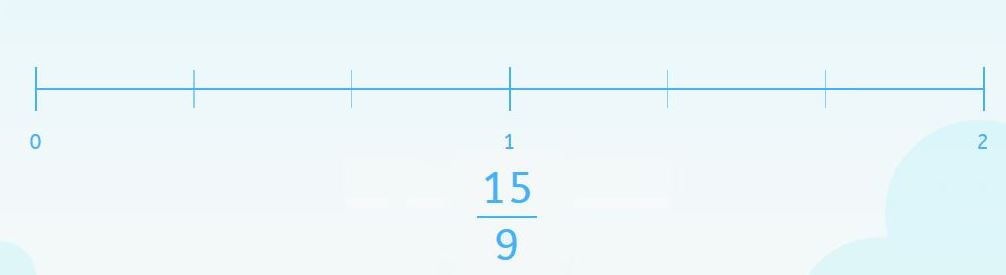This example shows that each unit on the number line has 3 divisions and the denominator of the fraction is 9. In order to have them match, we can simplify the fraction and obtain an equivalent fraction.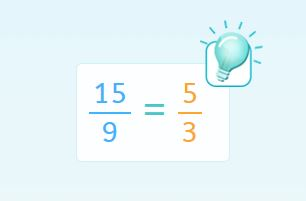In this case, the marker will be placed in the fifth position on the number line.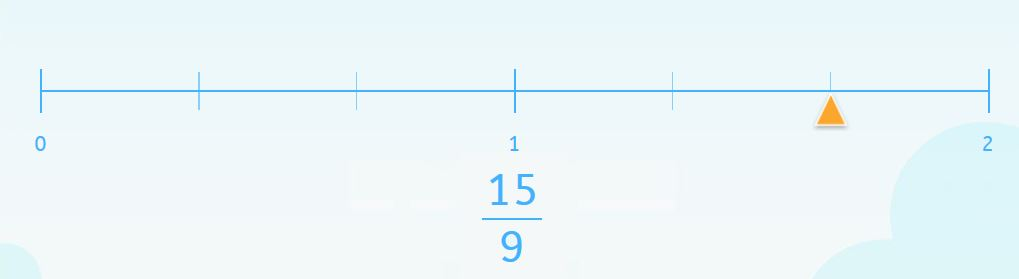#### Equivalent Fractions on a Number Line that Does Not Begin with Zero

And what if we make these activities a bit more complicated? Let’s take either of the two previous examples. But in this case, we’ll have to represent the fraction on a number line where the unit does not have the same number of divisions as the denominator and does not begin with zero.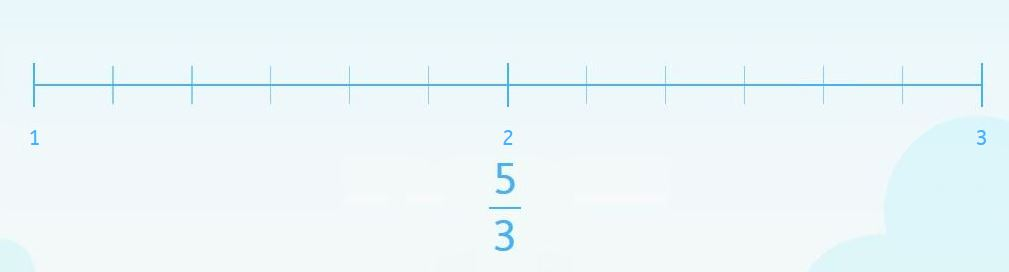This example is similar to the first. Therefore, we know that we have to look for an equivalent fraction by amplifying and the marker will be in the tenth position.But in the first example, the line did not begin with one. So in this case, we have to imagine that there’s a previous unit. That is where you should start counting from in order to place the marker correctly.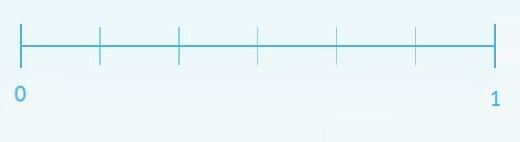And keep counting from there…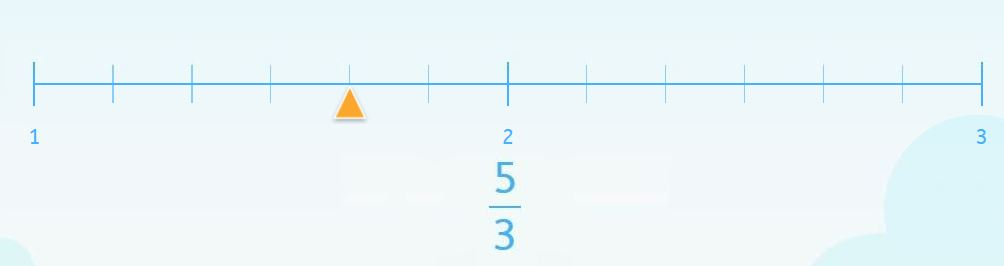If you would like to practice more equivalent fractions, number line activities, amplification, simplification and other elementary math content adapted to your level, register with Smartick and try it for free.